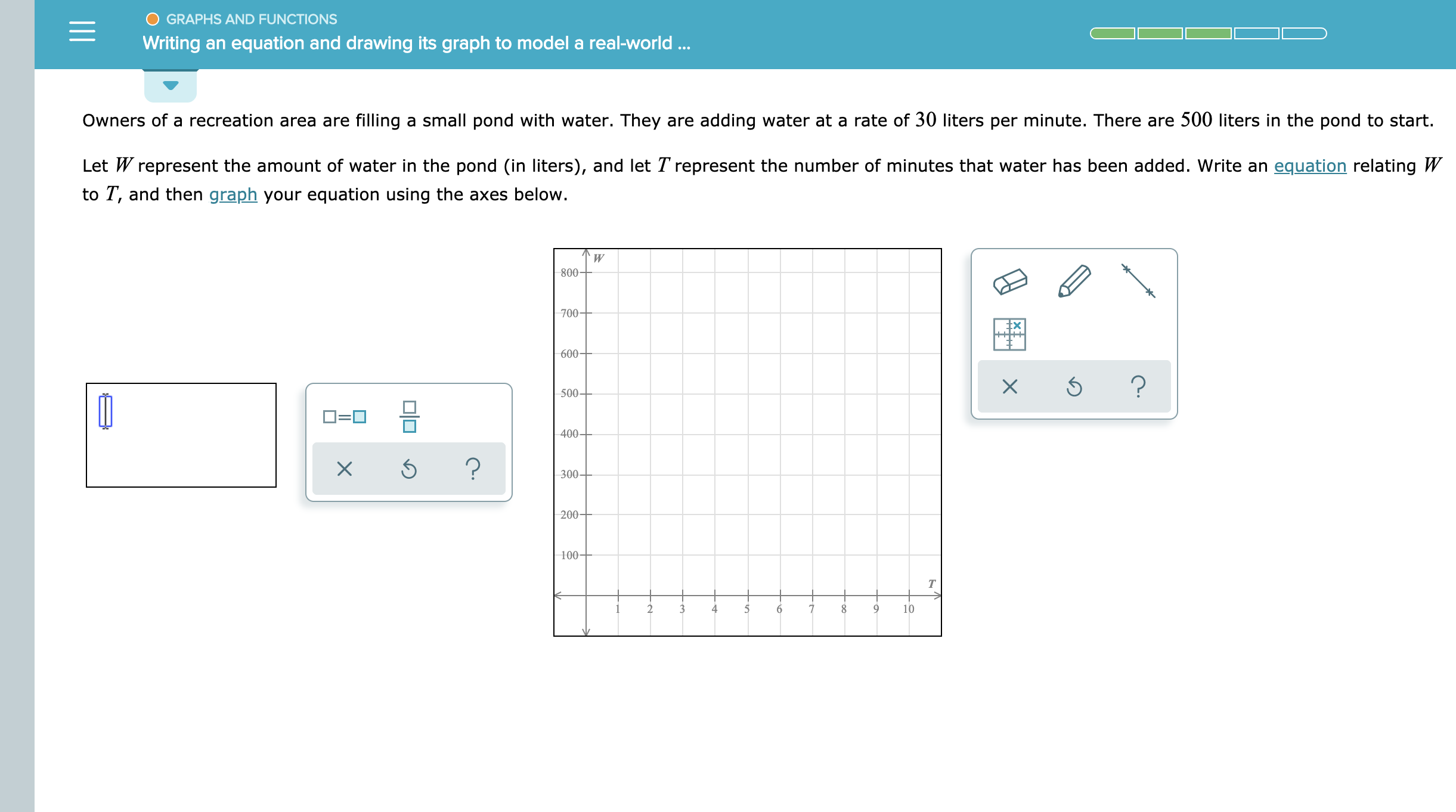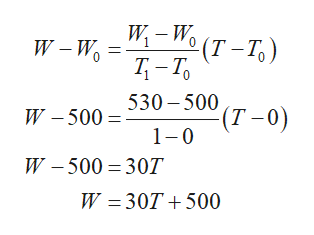# GRAPHS AND FUNCTIONSWriting an equation and drawing its graph to model a real-world...Owners of a recreation area are filling a small pond with water. They are adding water at a rate of 30 liters per minute. There are 500 liters in the pond to start.Let W represent the amount of water in the pond (in liters), and let T represent the number of minutes that water has been added. Write an equation relating Wto T, and then graph your equation using the axes below.W800-700600?500400-?300200-100т391046X

Question

See attachmenthelp_outlineImage TranscriptioncloseGRAPHS AND FUNCTIONS Writing an equation and drawing its graph to model a real-world... Owners of a recreation area are filling a small pond with water. They are adding water at a rate of 30 liters per minute. There are 500 liters in the pond to start. Let W represent the amount of water in the pond (in liters), and let T represent the number of minutes that water has been added. Write an equation relating W to T, and then graph your equation using the axes below. W 800- 700 600 ? 500 400- ? 300 200- 100 т 3 9 10 4 6 X fullscreen
check_circleExpert Solution
Step 1

Let W represents the amount of water in pond in liters and T represents the number of minutes that water has been added,

Since, initially there was 500 liters water in pond, therefore

At T=0, W=500

And since, the water is being added 30 liters per minute,

Therefore,

At T=1, W=500+30=530

Step 2

So, now take two points (0,500) and (1,530) and us...help_outlineImage TranscriptioncloseW-Wo (T-To) W-Wo T-To W -500530 -500 (T-0) 1 0 W-500 30T W 30T 500 fullscreen

### Want to see the full answer?

See Solution

#### Want to see this answer and more?

Solutions are written by subject experts who are available 24/7. Questions are typically answered within 1 hour*

See Solution
*Response times may vary by subject and question
Tagged in

### Functions